# K-means Clustering in Python

K-means clustering is a clustering algorithm that aims to partition $n$ observations into $k$ clusters.

There are 3 steps:

• Initialisation – K initial “means” (centroids) are generated at random
• Assignment – K clusters are created by associating each observation with the nearest centroid
• Update – The centroid of the clusters becomes the new mean

Assignment and Update are repeated iteratively until convergence

The end result is that the sum of squared errors is minimised between points and their respective centroids.

We’ll do this manually first, then show how it’s done using scikit-learn

Let’s view it in action using k=3:

In :
## Initialisation

import pandas as pd
import numpy as np
import matplotlib.pyplot as plt
%matplotlib inline

df = pd.DataFrame({
'x': [12, 20, 28, 18, 29, 33, 24, 45, 45, 52, 51, 52, 55, 53, 55, 61, 64, 69, 72],
'y': [39, 36, 30, 52, 54, 46, 55, 59, 63, 70, 66, 63, 58, 23, 14, 8, 19, 7, 24]
})

np.random.seed(200)
k = 3
# centroids[i] = [x, y]
centroids = {
i+1: [np.random.randint(0, 80), np.random.randint(0, 80)]
for i in range(k)
}

fig = plt.figure(figsize=(5, 5))
plt.scatter(df['x'], df['y'], color='k')
colmap = {1: 'r', 2: 'g', 3: 'b'}
for i in centroids.keys():
plt.scatter(*centroids[i], color=colmap[i])
plt.xlim(0, 80)
plt.ylim(0, 80)
plt.show()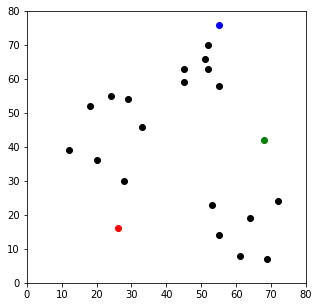In :
## Assignment Stage

def assignment(df, centroids):
for i in centroids.keys():
# sqrt((x1 - x2)^2 - (y1 - y2)^2)
df['distance_from_{}'.format(i)] = (
np.sqrt(
(df['x'] - centroids[i]) ** 2
+ (df['y'] - centroids[i]) ** 2
)
)
centroid_distance_cols = ['distance_from_{}'.format(i) for i in centroids.keys()]
df['closest'] = df.loc[:, centroid_distance_cols].idxmin(axis=1)
df['closest'] = df['closest'].map(lambda x: int(x.lstrip('distance_from_')))
df['color'] = df['closest'].map(lambda x: colmap[x])
return df

df = assignment(df, centroids)

fig = plt.figure(figsize=(5, 5))
plt.scatter(df['x'], df['y'], color=df['color'], alpha=0.5, edgecolor='k')
for i in centroids.keys():
plt.scatter(*centroids[i], color=colmap[i])
plt.xlim(0, 80)
plt.ylim(0, 80)
plt.show()

    x   y  distance_from_1  distance_from_2  distance_from_3  closest color
0  12  39        26.925824        56.080300        56.727418        1     r
1  20  36        20.880613        48.373546        53.150729        1     r
2  28  30        14.142136        41.761226        53.338541        1     r
3  18  52        36.878178        50.990195        44.102154        1     r
4  29  54        38.118237        40.804412        34.058773        3     b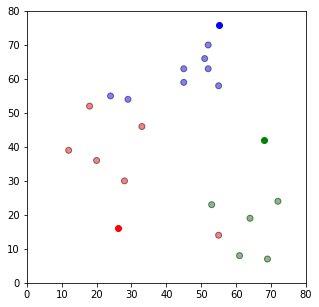In :
## Update Stage

import copy

old_centroids = copy.deepcopy(centroids)

def update(k):
for i in centroids.keys():
centroids[i] = np.mean(df[df['closest'] == i]['x'])
centroids[i] = np.mean(df[df['closest'] == i]['y'])
return k

centroids = update(centroids)

fig = plt.figure(figsize=(5, 5))
ax = plt.axes()
plt.scatter(df['x'], df['y'], color=df['color'], alpha=0.5, edgecolor='k')
for i in centroids.keys():
plt.scatter(*centroids[i], color=colmap[i])
plt.xlim(0, 80)
plt.ylim(0, 80)
for i in old_centroids.keys():
old_x = old_centroids[i]
old_y = old_centroids[i]
dx = (centroids[i] - old_centroids[i]) * 0.75
dy = (centroids[i] - old_centroids[i]) * 0.75
plt.show()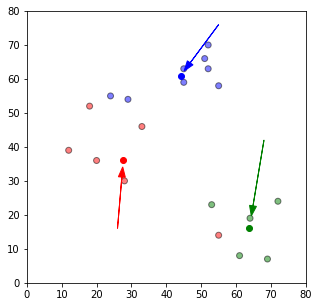In :
## Repeat Assigment Stage

df = assignment(df, centroids)

# Plot results
fig = plt.figure(figsize=(5, 5))
plt.scatter(df['x'], df['y'], color=df['color'], alpha=0.5, edgecolor='k')
for i in centroids.keys():
plt.scatter(*centroids[i], color=colmap[i])
plt.xlim(0, 80)
plt.ylim(0, 80)
plt.show()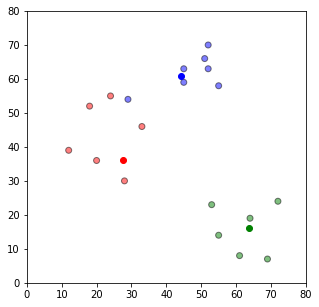Note that one of the reds is now green and one of the blues is now red.

We are getting closer.

We now repeat until there are no changes to any of the clusters.

In :
# Continue until all assigned categories don't change any more
while True:
closest_centroids = df['closest'].copy(deep=True)
centroids = update(centroids)
df = assignment(df, centroids)
if closest_centroids.equals(df['closest']):
break

fig = plt.figure(figsize=(5, 5))
plt.scatter(df['x'], df['y'], color=df['color'], alpha=0.5, edgecolor='k')
for i in centroids.keys():
plt.scatter(*centroids[i], color=colmap[i])
plt.xlim(0, 80)
plt.ylim(0, 80)
plt.show()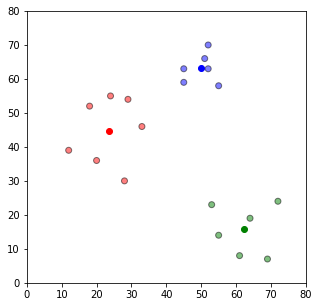So we have 3 clear clusters with 3 means at the centre of these clusters.

We will now repeat the above using scikit-learn, we first fit to our data

In :
df = pd.DataFrame({
'x': [12, 20, 28, 18, 29, 33, 24, 45, 45, 52, 51, 52, 55, 53, 55, 61, 64, 69, 72],
'y': [39, 36, 30, 52, 54, 46, 55, 59, 63, 70, 66, 63, 58, 23, 14, 8, 19, 7, 24]
})

from sklearn.cluster import KMeans

kmeans = KMeans(n_clusters=3)
kmeans.fit(df)

Out:
KMeans(algorithm='auto', copy_x=True, init='k-means++', max_iter=300,
n_clusters=3, n_init=10, n_jobs=1, precompute_distances='auto',
random_state=None, tol=0.0001, verbose=0)

Then we learn the labels

In :
labels = kmeans.predict(df)
centroids = kmeans.cluster_centers_

In :
fig = plt.figure(figsize=(5, 5))

colors = map(lambda x: colmap[x+1], labels)

plt.scatter(df['x'], df['y'], color=colors, alpha=0.5, edgecolor='k')
for idx, centroid in enumerate(centroids):
plt.scatter(*centroid, color=colmap[idx+1])
plt.xlim(0, 80)
plt.ylim(0, 80)
plt.show()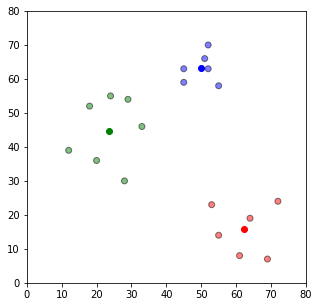We get the exact same result, albeit with the colours in a different order.

Some things to take note of though:

• k-means clustering is very sensitive to scale due to its reliance on Euclidean distance so be sure to normalize data if there are likely to be scaling problems.
• If there are some symmetries in your data, some of the labels may be mis-labelled
• It is recommended to do the same k-means with different initial centroids and take the most common label.